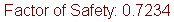Join one of our upcoming courses taking place around the world! Explore now

# Combinations Analysis Results

The main result from an SWedge Combinations analysis is the Minimum Factor of Safety Wedge. This is the wedge with the minimum factor of safety, of all wedges computed by the Combination Analysis.

The minimum factor of safety (FS) is displayed at the top of the screen, and a summary of results for the minimum factor of safety wedge is displayed in the Sidebar.The results for ALL wedges computed by the Combinations Analysis, are also available and can be plotted on Histograms, Scatter plots, Stereonet etc. In general, the analysis output from an SWedge Combination Analysis is similar to the analysis output from an SWedge Probabilistic Analysis. However, there are some important differences which are noted below.

## Combination Analysis versus Probabilistic Analysis

The Combination Analysis option in SWedge is NOT, strictly speaking, a Probabilistic Analysis. Therefore it is important to note the following differences.

• A Probability of Failure is NOT reported after a Combination Analysis. However, if desired, a Probability of Failure can be calculated by dividing the number of failed wedges by the total number of joint combinations. The applicability of a Probability of Failure calculated from a Combination analysis, depends on the nature of the input data (e.g. was the analysis computed for a single global orientation file or two discrete joint sets?). The interpretation of results in this regard is the responsibility of the user.
• Similarly, a Mean Wedge is also NOT defined for a Combination Analysis, because there is no explicitly defined mean joint orientation for a Combination analysis.
• Cumulative distribution plots are NOT available for a Combination Analysis, as cumulative plots are only applicable for Probabilistic analysis.

In most other respects, the analysis output from a Combination Analysis is similar to the Probabilistic analysis output. For information see the following topics (in the Probabilistic analysis section):